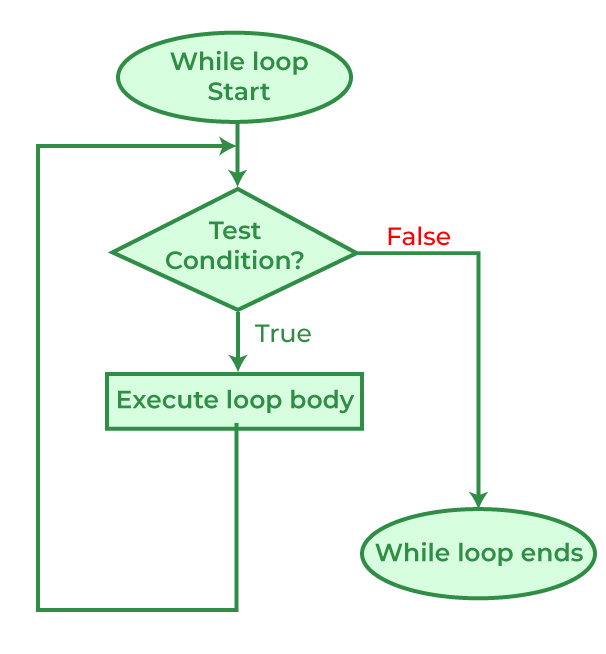# while loop in C

The while Loop is an entry-controlled loop in C programming language. This loop can be used to iterate a part of code while the given condition remains true.

## Syntax

The while loop syntax is as follows:

```while (test expression)
{
// body consisting of multiple statements
}```

## Example

The below example shows how to use a while loop in a C program

## C

 `// C program to demonstrate while loop` `#include `   `int` `main()` `{` `    ``// Initialization of loop variable` `    ``int` `i = 0;`   `    ``// setting test expression as (i < 5), means the loop` `    ``// will execute till i is less than 5` `    ``while` `(i < 5) {`   `        ``// loop statements` `        ``printf``(``"GeeksforGeeks\n"``);`   `        ``// updating the loop variable` `        ``i++;` `    ``}` `    ``return` `0;` `}`

Output

```GeeksforGeeks
GeeksforGeeks
GeeksforGeeks
GeeksforGeeks
GeeksforGeeks```

## while Loop Structure

The while loop works by following a very structured top-down approach that can be divided into the following parts:

1. Initialization: In this step, we initialize the loop variable to some initial value. Initialization is not part of while loop syntax but it is essential when we are using some variable in the test expression

2.  Conditional Statement: This is one of the most crucial steps as it decides whether the block in the while loop code will execute. The while loop body will be executed if and only the test condition defined in the conditional statement is true.

3. Body: It is the actual set of statements that will be executed till the specified condition is true. It is generally enclosed inside { } braces.

4. Updation: It is an expression that updates the value of the loop variable in each iteration. It is also not part of the syntax but we have to define it explicitly in the body of the loop.

## Flowchart of while loop in C## Working of while Loop

We can understand the working of the while loop by looking at the above flowchart:

1. STEP 1: When the program first comes to the loop, the test condition will be evaluated.

2. STEP 2A: If the test condition is false, the body of the loop will be skipped program will continue.

3. STEP 2B: If the expression evaluates to true, the body of the loop will be executed.

4. STEP 3:  After executing the body, the program control will go to STEP 1. This process will continue till the test expression is true.

## Infinite while loop

An infinite while loop is created when the given condition is always true. It is encountered by programmers in when:

• The test condition is incorrect.
• Updation statement not present.

## C

 `// C program to demonstrate an infinite while loop` `#include `   `int` `main()` `{` `    ``// Initialization` `    ``int` `gfg1 = 1;` `    ``int` `gfg2 = 1;`   `    ``// 'gfg1' is the Check/Test statement, which means that` `    ``// the while loop will iterate till the conditions` `    ``// satiate` `    ``while` `(gfg1 < 10) {`   `        ``// 'gfg2' is the body statements` `        ``gfg2 = gfg2 + 1;` `        ``printf``(``"GeeksforGeeks to Infinity"``);` `    ``}` `    ``// Return statement to tell that everything executed` `    ``// safely` `    ``return` `0;` `}`

Output

```GeeksforGeeks to Infinity
GeeksforGeeks to Infinity
GeeksforGeeks to Infinity
.........................```

As seen in the above example, the loop will continue till infinite because the loop variable will always remain the same resulting in the condition that is always true.

## Important Points

• It is an entry-controlled loop.
• It runs the block of statements till the conditions are satiated, once the conditions are not satisfied it will terminate.
• Its workflow is firstly it checks the condition and then executes the body. Hence, a type of pre-tested loop.
• This loop is generally preferred over for loop when the number of iterations is unknown.

Whether you're preparing for your first job interview or aiming to upskill in this ever-evolving tech landscape, GeeksforGeeks Courses are your key to success. We provide top-quality content at affordable prices, all geared towards accelerating your growth in a time-bound manner. Join the millions we've already empowered, and we're here to do the same for you. Don't miss out - check it out now!

Previous
Next